# Title 40

## SECTION 89.418

### 89.418 Raw emission sampling calculations.

§ 89.418 Raw emission sampling calculations.

(a) The final test results shall be derived through the steps described in this section.

(b) The exhaust gas flow rate GEXHW and VEXHW shall be determined for each mode.

(1) For measurements using the mass flow method, see § 89.416(a).

(2) For measurements using the fuel consumption and exhaust gas concentrations method, use the following equations: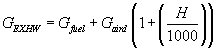Where: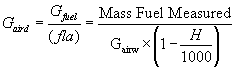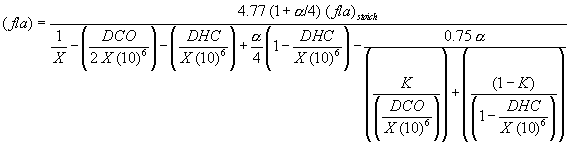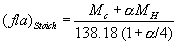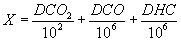K = 3.5

(3) Humidity values may be calculated from either one of the following equations: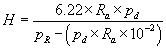or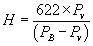(c) When applying GEXHW, the measured “dry” concentration shall be corrected to a wet basis, if not already measured on a wet basis. This section is applicable only for measurements made on raw exhaust gas. Correction to a wet basis shall be according to the following formula:

ConcWET = Kw × ConcDRY Where: KW is determined according to the equations in paragraph (c)(1) or (c)(2) of this section.

(1) For measurements using the mass flow method (see § 89.416(a)):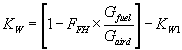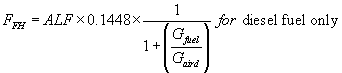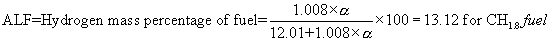α = H/C mole ratio of the fuel.

(2) For measurements using the fuel consumption and exhaust gas concentrations method (see § 89.416(b)):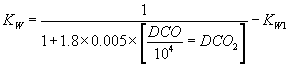Where: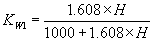(d) As the NOX emission depends on intake air conditions, the NOX concentration shall be corrected for intake air temperature and humidity with the factor Kh given in the following formula. For engines operating on alternative combustion cycles, other correction formulas may be used if they can be justified or validated. The formula follows: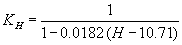(e) The pollutant mass flow for each mode shall be calculated as follows:

Gas mass = u × Gas conc. × GEXHW Gas mass = v × Gas conc. × VEXHD Gas mass = w × Gas conc. × VEXHW

The coefficients u (wet), v (dry), and w (wet) are to be used according to the following table:

Gas u v w conc.
NOX 0.001587 0.00205 0.00205 ppm.
CO 0.000966 0.00125 0.00125 ppm.
HC 0.000478 0.000618 ppm.
CO2 15.19 19.64 19.64 percent.

Note: The given coefficients u, v, and w are calculated for 273.15 °K (0 °C) and 101.3 kPa. In cases where the reference conditions vary from those stated, an error may occur in the calculations.

(f) The following equations may be used to calculate the coefficients u, v, and w in paragraph (e) of this section for other conditions of temperature and pressure:

(1) For the calculation of u, v, and w for NOX (as NO2), CO, HC (in paragraph (e) of this section as CH1.80), CO2, and O2:

Where: w = 4.4615.10−5 × M if conc. in ppm w = 4.4615.10−1 × M if conc. in percent v = w u = w/ρAir M = Molecular weight ρAir = Density of dry air at 273.15 °K (0 °C), 101.3 kPa = 1.293 kg/m 3

(2) For real gases at 273.15 °K (0 °C) and 101.3 kPa: For the calculation of u, v, and w

w = gas × 10−6 if conc. in ppm v = w u = w/pAir pGas = Density of measured gas at 0 °C, 101.3 kPas in g/m 3

(3) General formulas for the calculation of concentrations at temperature (designated as T) and pressure (designated as p):

- for ideal gases  - for real gases  with: 1% = 10 4 ppm M = Molecular weight in g/Mo1 Mv = Molecular Volume = 22.414 × 10−3 m 3/Mol for ideal gases T = reference temperature 273.15 K p = reference pressure 101.3 kPa T = Temperature in °C p = pressure in kPa pGas = Density of the measured gas at 0 °C, 101.3 kPa Conc. = Gas concentration

(g)(1) The emission shall be calculated for all individual components in the following way where power at idle is equal to zero: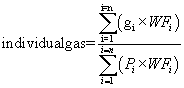(2) The weighting factors and the number of modes (n) used in the calculation in paragraph (g)(1) of this section are according to § 89.410.

[59 FR 31335, June 17, 1994. Redesignated and amended at 63 FR 56996, 57017, Oct. 23, 1998]